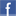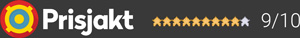#### Inga produkter tillagda!

Lägg en produkt i varukorgen så ser det lite roligare ut här ;)

#### Varukorg

0 kr# VincentDAC-7

16 990 kr/stVälj utförande
16 990 kr
16 990 kr
Leverans 2-5 dagar ()
Fri frakt!

## Fler bilder

Superläcker balanserad RÖR-DAC från Vincent.

Digital/Analogue-converter for the highest demands. Totally six different inputs are available working with a sampling rate up to 32bit and support DSD files (no MQA). The analogue part is working fully balancend and has a tube output stage.

Technical Specifications

Sampling Rate Coaxial, Optical, AES: 24 bit / 192 kHz (DSD:64)
Sampling Rate USB: 32 bit / 384 kHz (DSD:256)
Frequency Response: 20 Hz – 20 kHz +/- 0.5 dB, 20 Hz – 50 kHz +/-2 dB
Harmonic Distortion: < 0.0004 %
Signal-Noise Ratio: > 95 dB
Dynamic Range: > 100 dB
Audio Output Voltage: 2.5 V
Channel Separation: > 90 dB
Max. Power Consumption: 35 W
Inputs: 1 x Headphone Jack 6.3 mm, 2 x Coaxial, 2 x Optical, 1 x USB, 1 x AES
Outputs: 1 x Stereo XLR, 1 x Stereo RCA
Tubes: 1 x 6Z4, 2 x 12AU7
Playable Formats: MP3, WMA, AAC, AAC+, ALAC, FLAC, APE, WAC, DSD
Colour: Black / Silver
Weight: 6.5 kg
Dimensions (WxHxD): 430 x 95 x 360 mm

Garanti i 24 månaderDela på Facebook

#### Frågor? Ring 031-241600eller maila //<![CDATA[ var l=new Array(); l = '>'; l = 'a'; l = '/'; l = '<'; l = '|109'; l = '|111'; l = '|99'; l = '|46'; l = '|110'; l = '|114'; l = '|97'; l = '|108'; l = '|108'; l = '|97'; l = '|107'; l = '|100'; l = '|117'; l = '|106'; l = '|108'; l = '|64'; l = '|111'; l = '|102'; l = '|110'; l = '|105'; l = '>'; l = '"'; l = '|109'; l = '|111'; l = '|99'; l = '|46'; l = '|110'; l = '|114'; l = '|97'; l = '|108'; l = '|108'; l = '|97'; l = '|107'; l = '|100'; l = '|117'; l = '|106'; l = '|108'; l = '|64'; l = '|111'; l = '|102'; l = '|110'; l = '|105'; l = ':'; l = 'o'; l = 't'; l = 'l'; l = 'i'; l = 'a'; l = 'm'; l = '"'; l = '='; l = 'f'; l = 'e'; l = 'r'; l = 'h'; l = ' '; l = 'a'; l = '<'; for (var i = l.length-1; i >= 0; i=i-1) { if (l[i].substring(0, 1) === '|') document.write("&#"+unescape(l[i].substring(1))+";"); else document.write(unescape(l[i])); } //]]>